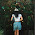## Fili's Proportion Problems

Proportion Problems
1) Sam can do 12 math problems in 45 minutes. How long will it take for Sam to finish 20 math problems

Let x be the minutes for 20 math problems
Steps: Word Ratio, Ratio table, Find x

Sam can do 20 math problems in 75 minutes

2) Sam is trying to make lemonade. He can make lemonade with 6 lemons and 8 cups of water. How many lemons does Sam need if he has 24 cups of water.

Let x be the amount of lemons needed for 24 cups of water
Steps: Word Ratio, Ratio Table, Find x
Sam needs a total of 18 lemons for 24 cups of water
7:16 PM

#### 1 comment :

1.Fili, where is your third question? And we were also suppose to add pics just like our rate and unit rate problem (including the link). But you did a good job in the two questions! yay *thumbs up emoji* :)